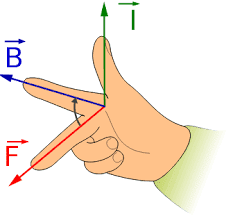# Direction of electric field

## Homework Statement

beam of protons moving in +x direction at speed v
electric field is perpendicular to magnetic field
magnetic field in +y direction
no deflection
What direction/sign has the electric field?

## Homework Equations

not looking for numerical solutions

## The Attempt at a Solution

There's no deflection, the magnetic and electric force have same magnitude, but opposing directions, correct?
The magnetic force is perpendicular to the magnetic field, while the electric force is in the direction of the electric field.
How do I then find the direction of the electric field?
+x and -x are perpendicular to +y. Let's say the magnetic force has a magnitude of 5, the electric force would be -5. Is the direction of the magnetic force -x, i.e. magnitude of -5 and thus the electric force a direction of +x, i.e. magnitude of 5?

blue_leaf77
Homework Helper
There's no deflection, the magnetic and electric force have same magnitude, but opposing directions, correct?
Yes.
First you have to find out what can be found out first. In this case, the magnetic force, because you know the directions of the protons' speed and the magnetic field. The magnetic force exerted on a charged particle moving with a velocity ##\mathbf{v}## in a uniform magnetic field ##\mathbf{B}## is given by ##\mathbf{F}_M=q\mathbf{v}\times \mathbf{B}##. What is then the direction of the magnetic force in this problem?

•hmaier
andrevdh
Homework Helper
What direction is the electric field in according to that statement?

•hmaier
my reply got deleted as I wasn't logged in properly...

the electric field, like the magnetic force should be perpendicular to the magnetic field.
I used your formula blue_leaf77 for the calculations. I think I actually need to use the right hand rule to determine the direction?
That gave me a direction pointint out of the paper given the usual x-y-z coordinate system a -z direction for the magnetic force, which whould mean that electric force would be +z.
I hope, I'm not getting totally off track here!

blue_leaf77
Homework Helper
a -z direction
Almost correct. The velocity of the particle is in the x direction and the magnetic field is in the y direction. Using the right hand rule, which direction do you get for the magnetic force?

•hmaier
ok, so, index finger(particles) on the +x-axis, thumb (magnetic field) on +y-axis, so magnetic force is actually "into the paper", i.e. the positive z-axis?
I will need some sort of hand massage after this ;)

blue_leaf77
Homework Helper
I am rather confused with the way you define the directions, I think your definition is slightly different than mine. Anyway, you are correct, the magnetic force points to the positive z direction.
(By the way, I am using)

•hmaier
My friend provided me with the teaching materials from a course he took. The assignment of fingers is switched around. B is the middle finger, F thumb and I index finger.
As long as it yields the same result, there's no harm done!
Thanks a million for guiding me along so generously. I'm really enjoying diving into physics and maths again after so many years.